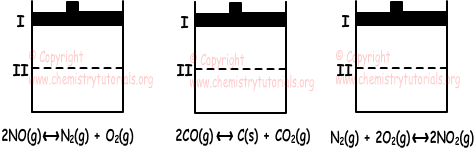# Factors Affecting Chemical Equilibrium

Factors Affecting Chemical Equilibrium

Concentration, temperature and pressure effect chemical equilibrium. We explain them in detail one by one. However, we first give you Le Chatelier’s principle of equilibrium;

" If one of the factors like temperature , pressure or concentration of system is changed then system react this change to conserve equilibrium."

1. Affect of Concentration on Equilibrium:

Adding or removing matters into reaction effect equilibrium. For example, adding reactants or removing products increase the yield of product. On the contrary, adding products or removing reactants increase yield of reactants. In other words, in first situation equilibrium shifts to the right and in second situation equilibrium shifts to the left. For instance;

H2(g) + I2(g) ↔ 2HI(g)

If we add H2 gas to the container, equilibrium shifts to the right and system decrease the concentration of H2. ( Le Chatelier’s principle)

2. Affect of Temperature on Equilibrium:

Heat must be given to system at equilibrium to increase temperature of it. This process gives different results in endothermic and exothermic reactions. For example;

H2(g) + I2(g) ↔ 2HI(g) + Heat

Reaction given above is exothermic. To keep equilibrium temperature must be constant. If heat is given to system, according to Le Chatelier’s principle system wants to decrease this temperature and equilibrium shift to the left or right. Equilibrium constant of this reaction;

Kc=[HI]2/([I2].[H2])

• In an endothermic reaction; increasing temperature shift equilibrium to the right and equilibrium constant increases.
• In an endothermic reaction; increasing temperature shift equilibrium to the left and equilibrium constant decreases.

3. Affect of Pressure on Equilibrium:

To talk about affect of pressure on equilibrium, at least one of the matters must be in gas phase. In other words, in a reaction consisting of liquid, aqueous, or solid there is no affect of pressure on equilibrium of this system.

• If one of the matters in container  under constant temperature and pressure is removed or added, pressure of the system changes. However, change in the concentration is taken into consideration not pressure.
• Temperature can be changed under constant volume. In this situation even if pressure changes, we consider changes in the temperature while finding equilibrium constant.
• In gas reactions, if there is no change in number of moles, then pressure do not effect equilibrium.

Example: Three container given below are in equilibrium with given reactions.If volumes of them decreased from point I to II, find in which container equilibrium shifts to the right.

Solution:

I. In first container, there is no change in the number of moles. Thus, pressure does not effect this reaction.

II. In second container, there is no change in the total number of moles. But, in this reaction moles of gas in this reaction decreases. So, equilibrium shifts to the right.

III. As you can see from the reaction, number of moles of decreases. Thus equilibrium shifts to the right.

Chemical Equilibrium Exams and Problem Solutions